Study P1 Mathematics Addition within 20 - Geniebook## Topic Recap:

Example 1:

Complete the equation:There are __________ candies altogether.

8

Solution: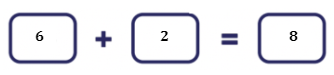There are 6 candies inside the container. There are also two candies that are not inside the container. In order to find how many candies there are altogether, we will need to add these numbers up.

Example 2:

How many glasses of juice are there altogether?1. 1
2. 6
3. 3
4. 7

(4) 7

Solution: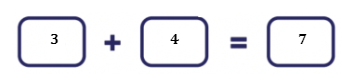There are 3 glasses on the tray. There are also four glasses that are not on the tray. In order to find how many glasses there are altogether, we will need to add these numbers up.

Example 3:

Draw to make 10 and fill in the blank.8 and __________ make 10.

2

Solution:

As we only have 8 stars now, we need to draw 2 more stars to form 10.

$$8 + 2 = 10$$

Example 4:

Which pair of numbers add up to 10?

1. 1 and 7
2. 6 and 2
3. 3 and 4
4. 9 and 1

(4) 9 and 1

Solution:

Let’s solve all the options.

1. $$1 + 7 = 8$$
2. $$6 + 2 = 8$$
3. $$3 + 4 = 7$$
4. $$9 + 1 = 10$$

Example 1:

Stephen has 11 chocolate milkshakes and 3 strawberry milkshakes. How many milkshakes does he have altogether?Stephen has __________ milkshakes altogether.

14

Solution: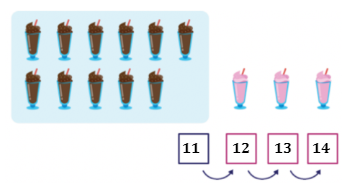There are 11 chocolate milkshakes  in the box.  Count on from 11:

12, 13, 14.

Question 1:How many ice-creams are there altogether?

$$8 + 6 =$$ __________

1. 12
2. 14
3. 16
4. 18

(2) 14

Solution: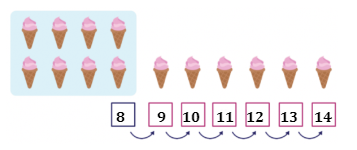There are 8 ice creams in the box. Count on from 8:

9, 10, 11, 12, 13, 14.

Question 2:

How many robots are there altogether?1. 14
2. 16
3. 18
4. 19

(4) 19

Solution:There are 12 robots in the box. Count on from 12:

13, 14, 15, 16, 17, 18, 19.

## Maths Concept: Addition Within 20 By Making 10

Example 1:

There are 5 doughnuts in a box. There are 7 doughnuts on a plate. How many doughnuts are there altogether?12

Solution:

$$5 + 7 =$$ __________

Step 1:

$$7 = 5 + 2$$

Step 2:

Make 10 first.

Step 3:

\begin{align}​ 5 + 5 &= 10 \\ 10 + 2 &= 12 \end{align}

Question 1:Fill in the blank with the correct answer:

$$6 + 9 =$$ __________

15

Solution:

Step 1:

$$9 = 4 + 5$$

Step 2:

Make 10 first.

Step 3:

\begin{align}​ 6 + 4 &= 10 \\ 10 + 5 &= 15 \end{align}

Question 2:

Fill in the blank with the correct answer.$$9 + 3 =$$ __________

1. 10
2. 12
3. 15
4. 17

(2) 12

Solution:

Step 1:

$$9 = 7 + 2$$

Step 2:

Make 10 first.

Step 3:

\begin{align}​ 3 + 7 &= 10 \\ 10 + 2 &= 12 \end{align}

Question 3:

Fill in the blank with the correct answer:

$$4 + 7 =$$ __________

1. 11
2. 12
3. 14
4. 17

(1) 11

Solution:

Step 1:

$$7 = 6 + 1$$

Step 2:

Make 10 first.

Step 3:

\begin{align}​ 6 + 4 &= 10 \\ 10 + 1 &= 11 \end{align}

Question 4:

Jane has 14 red pens. She also has 5 blue pens. How many pens does Jane have altogether?$$14 + 5 =$$ __________

19

Solution:

Step 1:

$$14 = 10 + 4$$

Step 2:

Step 3:

\begin{align}​ 4 + 5 &= 9 \\ 10 + 9 &= 19 \end{align}

Question 5:

Fill in the blank with the correct answer:$$18 = 10 \;+$$ __________

1. 7
2. 8
3. 10
4. 11

(2) 8

Solution: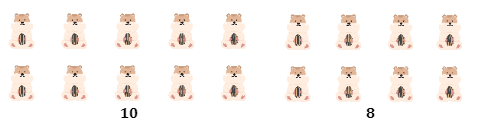Group the items into 10, then count the rest.

Question 6:

Fill in the blank with the correct answer:$$13 + 6 =$$ __________

1. 16
2. 17
3. 18
4. 19

(4) 19

Solution:

Separate into 10 and its ones, and add the ones.10                3                          6

Step 1:

$$13 = 10 + 3$$

Step 2:

$$3 + 6 = 9$$

Step 3:

$$10 + 9 = 19$$

Question 7:

Fill in the blank with the correct answer:$$15 + 5 =$$ __________

1. 10
2. 12
3. 17
4. 20

(4) 20

Solution: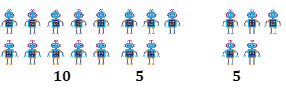Separate into 10 and its ones, and add the ones.

Step 1:

$$15 = 10 + 5$$

Step 2:

$$5 + 5 = 10$$

Step 3:

$$10 + 10 = 20$$

Question 8:

Fill in the blank with the correct answer:

1. 11
2. 17
3. 18
4. 19

(2) 17

Solution:

Step 1:

$$14 = 10 + 4$$

Step 2:

$$4 + 3 = 7$$

Step 3:

$$10 + 7 = 17$$

Question 9:

What is $$5 + 13$$?

1. 8
2. 10
3. 13
4. 18

(4) 18

Solution:

Step 1:

$$13 = 10 + 3$$

Step 2:

$$5 + 3 = 8$$

Step 3:

$$10 + 8 = 18$$

Question 10:

Which of the following shows the addition sentence that best represents the diagram below?1. $$10 + 8$$
2. $$11 + 6$$
3. $$11 + 8$$
4. $$12 + 6$$

(3) $$11 + 8$$

Solution:

There are 11 yellow planes and 8 blue planes. Therefore, the addition sentence will be $$11 + 8$$.

Question 11:

Which of the following expressions is not equal to 16?(4) $$12 + 5 = 17$$

Solution:

1.   $$8 + 8 = 16$$
2. $$10 + 6 = 16$$
3. $$15 + 1 = 16$$
4. $$12 + 5 = 17$$

Question 12:

Which of the following has the smallest answer?(4) $$12 + 1$$

Solution:

1.   $$9 + 9 = 18$$
2. $$11 + 5 = 16$$
3. $$10 + 4 = 14$$
4. $$12 + 1 = 13$$

Question 13:

Which of the following has the greatest answer?(1) $$1 + 18$$

Solution:

1. $$1 + 18 = 19$$
2. $$10 + 7 = 17$$
3.   $$3 + 9 = 12$$
4. $$11 + 6 = 17$$

Question 14:

Which three of the following numbers will form an addition equation?

13, 4, 7, 201. 13, 4, 7
2. 13, 7, 20
3. 4, 7, 20
4. 4, 13, 20

(2) 13, 7, 20

Solution:

Try each option.

$$13 + 7 = 20$$

 Continue Learning Numbers To 10 Picture Graphs 1 Shapes 1 Ordinal Numbers Subtraction Within 20 Addition Within 10 Addition And Subtraction Within 10 Numbers To 20 Addition Within 20 Numbers To 100 Addition Within 100PrimaryPrimary 1EnglishMaths
Numbers To 10
Picture Graphs 1
Shapes 1
Ordinal Numbers
Subtraction Within 20
Numbers To 20
Numbers To 100Primary 2Primary 3Primary 4Primary 5Primary 6SecondarySecondary 1Secondary 2Secondary 3Secondary 4